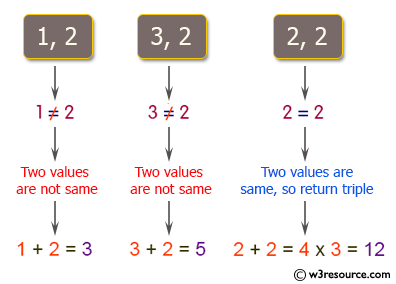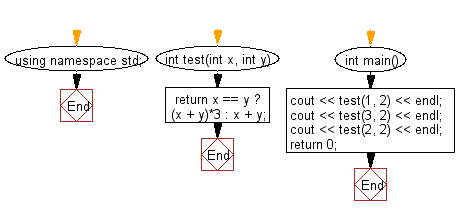﻿ C++ Exercises: Compute the sum of the two given integer values - w3resource# C++ Exercises: Compute the sum of the two given integer values

## C++ Basic Algorithm: Exercise-1 with Solution

Write a C++ program to compute the sum of the two given integer values. If the two values are the same, then return triple their sum.

Sample Solution:

C++ Code :

``````#include <iostream>

using namespace std;

int test(int x, int y)
{
return x == y ? (x + y)*3 : x + y;
}

int main()
{
cout << test(1, 2) << endl;
cout << test(3, 2) << endl;
cout << test(2, 2) << endl;
return 0;
}
``````

Sample Output:

```3
5
12
```

Pictorial Presentation:Flowchart:C++ Code Editor:

Contribute your code and comments through Disqus.

What is the difficulty level of this exercise?

﻿